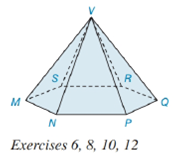Chapter 9.2, Problem 10E### Elementary Geometry for College St...

6th Edition
Daniel C. Alexander + 1 other
ISBN: 9781285195698

#### Solutions

Chapter
Section### Elementary Geometry for College St...

6th Edition
Daniel C. Alexander + 1 other
ISBN: 9781285195698
Textbook Problem
1 views

# Suppose that the base of the hexagonal pyramid in Exercise 6 has an area of 41.6 c m 2 and that of each lateral face has an area of 20 c m 2 . Find the total (surface) area of the pyramid.To determine

To find:

The total surface area of the given pyramid.

Explanation

Given:

The figure given below

and base of the hexagonal pyramid has an area of 41.6cm2 and each lateral face has an area of 20cm2.

Formula Used:

A pyramid is made by connecting a base to an apex or vertex. The base is flat with straight edges, no curves, hence, a polygon and all other faces are triangles.

A regular pyramid is a pyramid whose base is a regular polygon and whose lateral edges are all congruent.

The lateral faces are given by the triangular faces of the pyramid.

The total surface area T of a pyramid with lateral area A and base area B is given by

T=L+B.

The area of a square B of side a is given by

B=a2.

Calculation:

From the given figure

The apex is directly above the center of the base. Hence it is a right Pyramid and the lateral edges are congruent.

The lateral faces are congruent. Thus, a regular pyramid.

The number of lateral faces is given by the triangular faces of the pyramid i

### Still sussing out bartleby?

Check out a sample textbook solution.

See a sample solution

#### The Solution to Your Study Problems

Bartleby provides explanations to thousands of textbook problems written by our experts, many with advanced degrees!

Get Started